Premium

# 2.OA.A.1 Worksheets, Workbooks, Lesson Plans, and Games

#### CCSS.maths.CONTENT.2.OA.A.1

:
"Use addition and subtraction within 100 to solve one- and two-step word problems involving situations of adding to, taking from, putting together, taking apart, and comparing, with unknowns in all positions, e.g., by using drawings and equations with a symbol for the unknown number to represent the problem."

Our Take:The second grader can choose from a variety of different problem-solving strategies when solving one- and two-step addition and subtraction word problems. Some of these strategies may include base-ten drawing, open number line, or writing a number sentence. The computation difficulty increases in year three as students are challenged to add and subtract within 100.

These worksheets, games, and lesson plans can help students practise this Common Core State Standards skill.

## Worksheets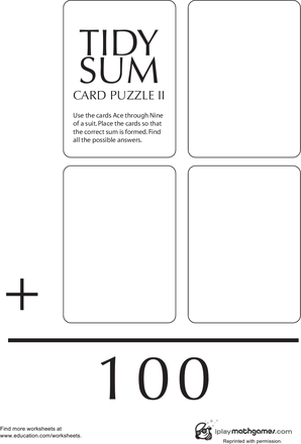Tidy Sum 100
Worksheet
Tidy Sum 100
Work on addition while finding sums of 100 using a deck of playing cards and this year two maths worksheet.
Year 3
Maths
Worksheet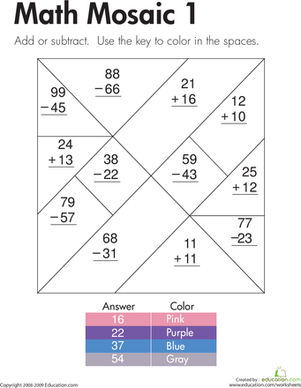Addition and Subtraction: maths Mosaic 1
Worksheet
Addition and Subtraction: maths Mosaic 1
After kids solve the two-digit addition and subtraction problems on this year three maths worksheet, they use the key to colour in the shapes on the mosaic.
Year 3
Maths
Worksheet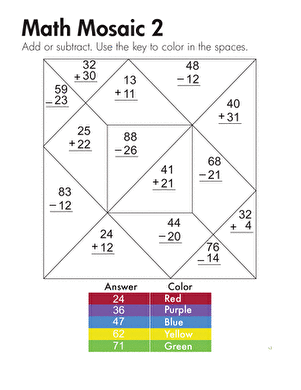Maths Mosaic
Worksheet
Maths Mosaic
After kids solve the two-digit addition and subtraction problems on this year three maths mosaic, they use the key to colour in the shapes.
Year 3
Maths
Worksheet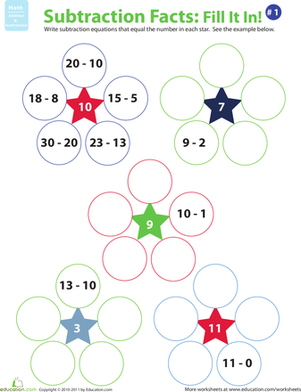Subtraction Equations
Worksheet
Subtraction Equations
Can your second grader come up with subtraction equations that equal the number in each star?
Year 3
Maths
Worksheet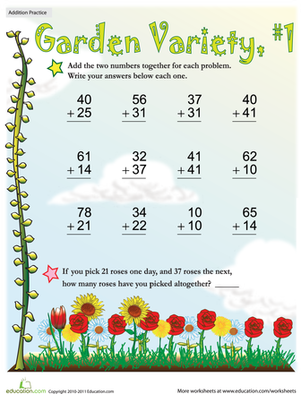Garden Variety Addition #1
Worksheet
Garden Variety Addition #1
Does your child need help with two-digit addition? This colorful flower-filled worksheet is a great way to give her the practise she needs.
Year 3
Worksheet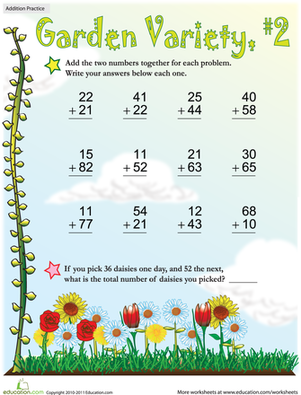Garden Variety Addition #2
Worksheet
Garden Variety Addition #2
Test your child's arithmetic skills with a worksheet on two-digit addition. He'll practise his addition facts know-how and flex his mental maths skills.
Year 3
Worksheet

## Lesson Plans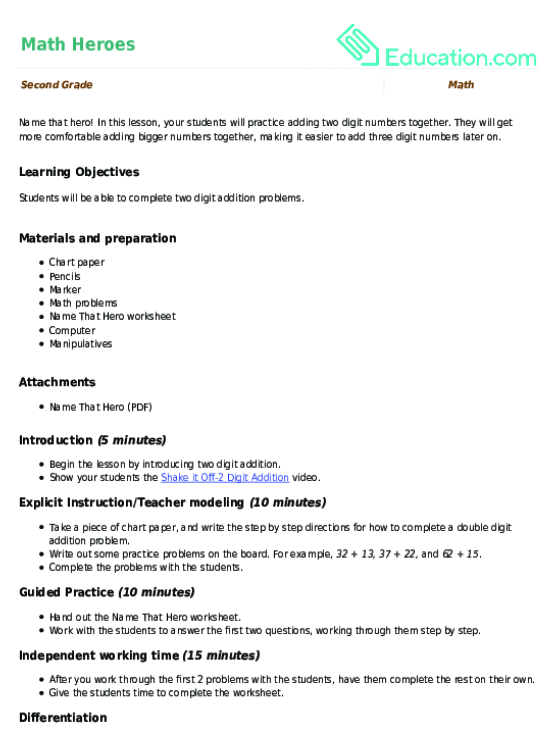Maths Heroes
Lesson plan
Maths Heroes
Name that hero! In this lesson, your students will practise adding two digit numbers together. They will get more comfortable adding bigger numbers together, making it easier to add three digit numbers later on.
Year 3
Maths
Lesson plan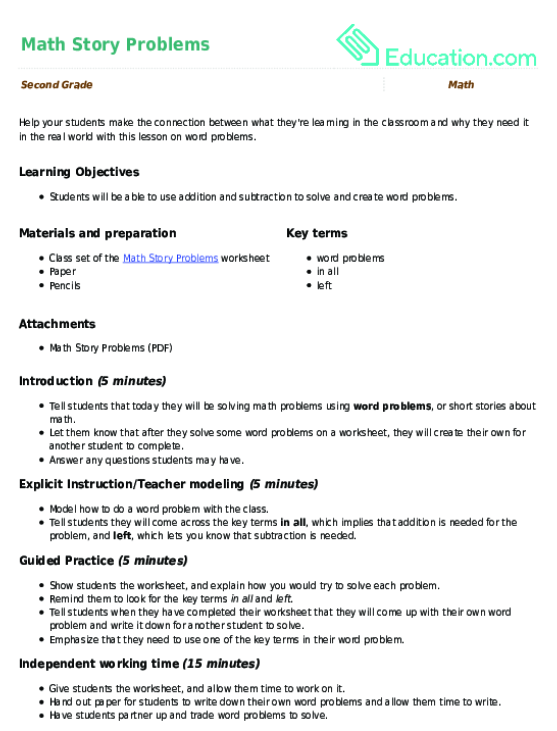Maths Story Problems
Lesson plan
Maths Story Problems
Help your students make the connection between what they're learning in the classroom and why they need it in the real world with this lesson on word problems.
Year 3
Maths
Lesson plan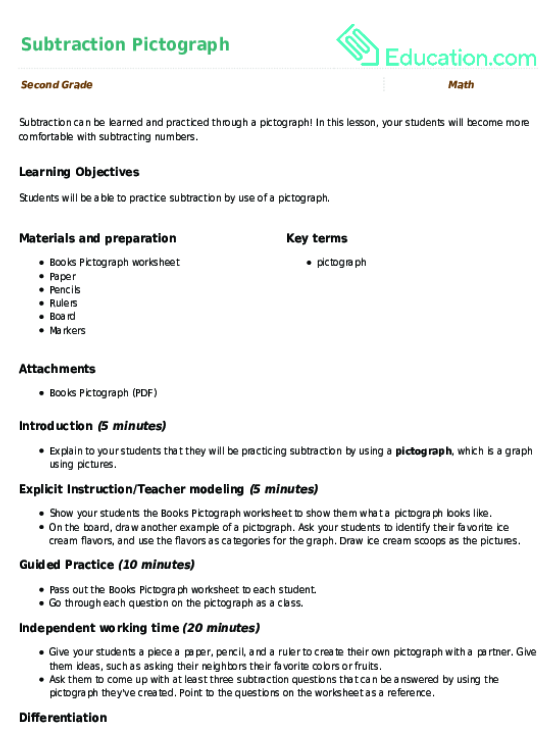Subtraction Pictograph
Lesson plan
Subtraction Pictograph
Subtraction can be learned and practiced through a pictograph! In this lesson, your students will become more comfortable with subtracting numbers.
Year 3
Maths
Lesson plan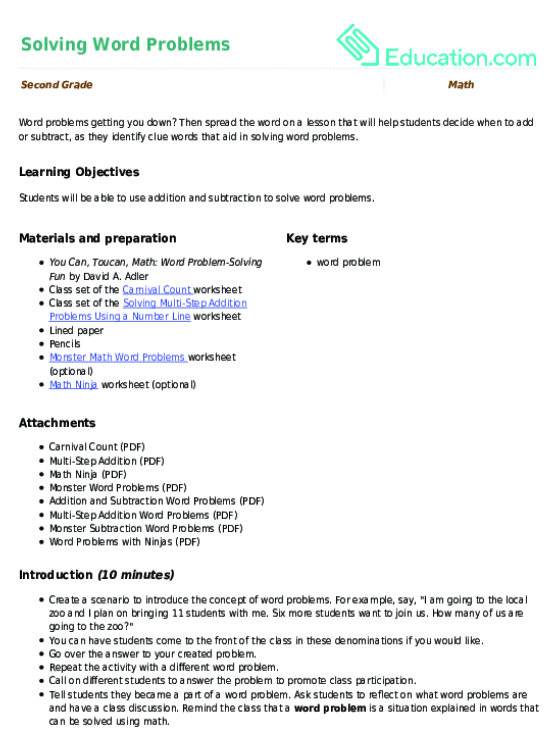Solving Word Problems
Lesson plan
Solving Word Problems
Word problems getting you down? Then spread the word on a lesson that will help students decide when to add or subtract, as they identify clue words that aid in solving word problems.
Year 3
Maths
Lesson plan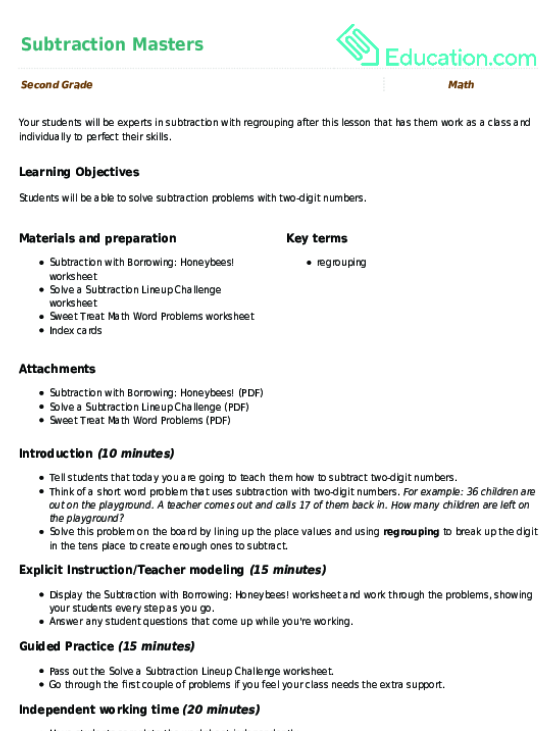Subtraction Masters
Lesson plan
Subtraction Masters
Your students will be experts in subtraction with regrouping after this lesson that has them work as a class and individually to perfect their skills.
Year 3
Maths
Lesson planMystery Addends: Representing Word Problems
Lesson plan
Mystery Addends: Representing Word Problems
Make a maths mystery! In this lesson, help your students understand the relationship between addition and subtraction and how a missing addend word problem is represented with a number sentence.
Year 2
Maths
Lesson plan

## Workbooks

No workbooks found for this common core node.

## Games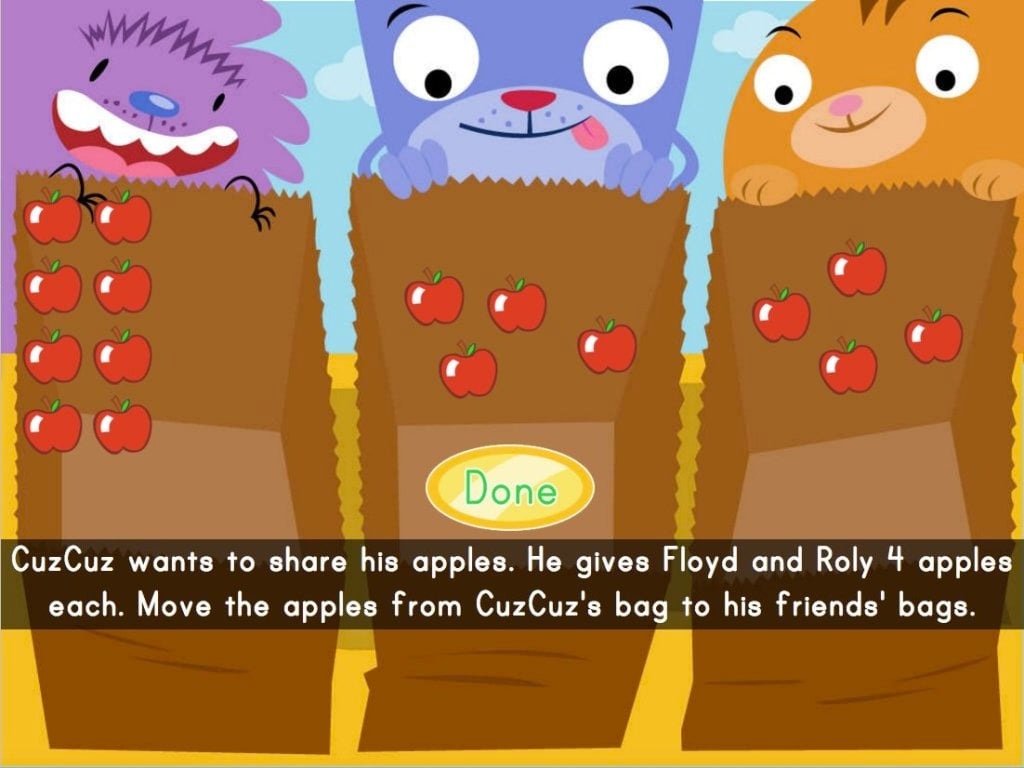Two-Step Subtraction
Game
Two-Step Subtraction
Kids subtract apples in two-step equations to find a target number.
Year 3
Maths
Game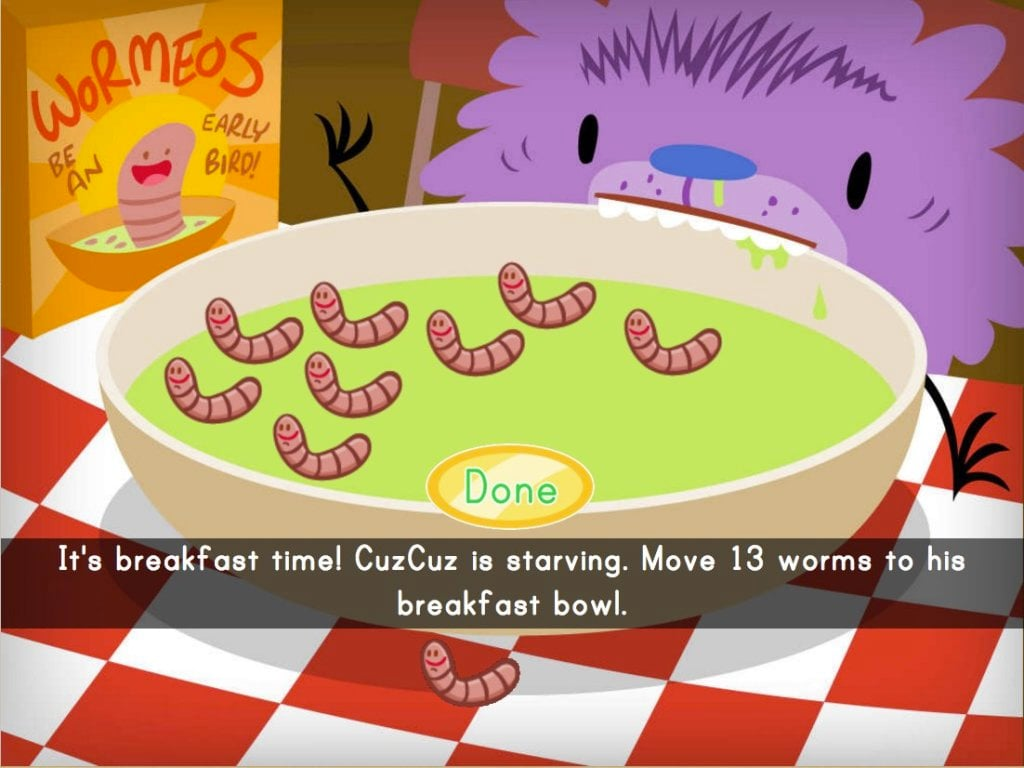Adding Worms with Cuz-Cuz
Game
Adding Worms with Cuz-Cuz
Kids practise adding small numbers in this silly addition game.
Year 3
Maths
Game

## Exercises

No exercises found for this common core node.

### Add to collection

Create new collection

0

### New Collection>

0Items

What could we do to improve Education.com?

Please note: Use the Contact Us link at the bottom of our website for account-specific questions or issues.

What would make you love Education.com?